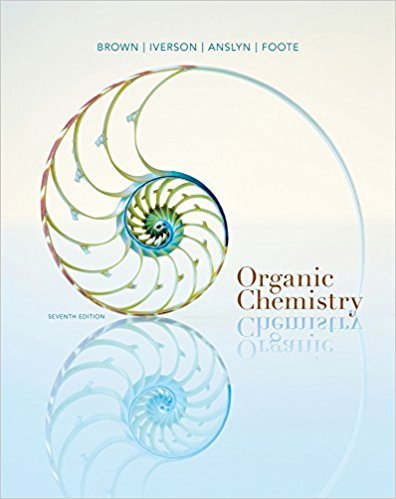×
Get Full Access to Organic Chemistry - 7 Edition - Chapter 20 - Problem 20.48
Get Full Access to Organic Chemistry - 7 Edition - Chapter 20 - Problem 20.48

×

# We showed in Figure 20.10 that a chairlike transition state for a [3,3]-sigmatropicISBN: 9781133952848 483

## Solution for problem 20.48 Chapter 20

Organic Chemistry | 7th Edition

• Textbook Solutions
• 2901 Step-by-step solutions solved by professors and subject experts
• Get 24/7 help from StudySoup virtual teaching assistantsOrganic Chemistry | 7th Edition

4 5 1 244 Reviews
20
0
Problem 20.48

We showed in Figure 20.10 that a chairlike transition state for a [3,3]-sigmatropic shift isallowed via frontier molecular orbital theory.(a) Write analogous pictures for a boatlike reaction geometry showing that this is alsoallowed.(b) Why are products from this reaction geometry formed to a much lower extent thanthose that proceed via a chairlike transition state?

Step-by-Step Solution:
Step 1 of 3

CHAPTER 14: CHEMICAL EQUILIBRIUM Dynamic Equilibrium  Important things at equilibrium o Forward rate= reverse rate : products are being created equally as fast as the reactants are being created o The concentrations of the reactants and products are not changing over time: the ratio between the concentrations of the reactants and products are equal over time o The concentration of the reactants and products are not necessarily equal: there might be more product than reactant, but the ratio comparing the two will remain constant The Reaction Quotient and Equilibrium Quotient  Law Mass of Action: o In general: aA + bB cC + dD *lower case letters represent the coefficients* o K= [C] [D] = [products] when the system is at equilibrium a b [A] [B] [reactants] K= equilibrium constant (K : coccentrations in mol/L or M) c d o Q= [C] [D] = [products] when the system is NOT at equilibrium [A] [B] [reactants] Q= reaction quotient ** K and Q have equal formulas but are used in different situations** o Example: For the equation N O 2(g4  2 NO (g), 2ind the equilibrium constant 2 K= [NO ]2 ** since 2O is the product and

Step 2 of 3

Step 3 of 3

## Discover and learn what students are asking

Chemistry: The Central Science : Liquids and Intermolecular Forces
?The phase diagram for neon is Use the phase diagram to answer the following

Unlock Textbook Solution